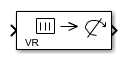# Rotation Matrix to VR Rotation

Convert rotation matrix to axis/angle rotation

• Library:
• Simulink 3D Animation / Utilities

•## Description

The Rotation Matrix to VR Rotation converts Rotation Matrix (defined columnwise as 3-by-3 matrix or as a 9-element column vector) into the Axis / Angle rotation representation used for defining rotations in VR.

## Ports

### Input

expand all

3D rotation, specified as a 3-by-3 columnwise-defined matrix, also known as a direction cosine matrix.

A representation of a three-dimensional spherical rotation as a 3-by-3 real, orthogonal matrix R: RTR = RRT = I, where I is the 3-by-3 identity and RT is the transpose of R. This matrix is also known as the direction cosine matrix (DCM). The DCM is the orientation of the object in space, relative to its parent node.

`$R=\left(\begin{array}{ccc}{R}_{11}& {R}_{12}& {R}_{13}\\ {R}_{21}& {R}_{22}& {R}_{23}\\ {R}_{31}& {R}_{32}& {R}_{33}\end{array}\right)=\left(\begin{array}{ccc}{R}_{xx}& {R}_{xy}& {R}_{xz}\\ {R}_{yx}& {R}_{yy}& {R}_{yz}\\ {R}_{zx}& {R}_{zy}& {R}_{zz}\end{array}\right)$`

Data Types: `single` | `double`

### Output

expand all

Output rotation, returned as a 4-element vector in axis/angle notation,. The first three elements specify the axis of rotation and the fourth element specifies the angle.

## Parameters

expand all

Input signal value is considered to be zero if it is equal to or lower than the value set in this parameter. By default, the parameter is set to ε = 1e-12.